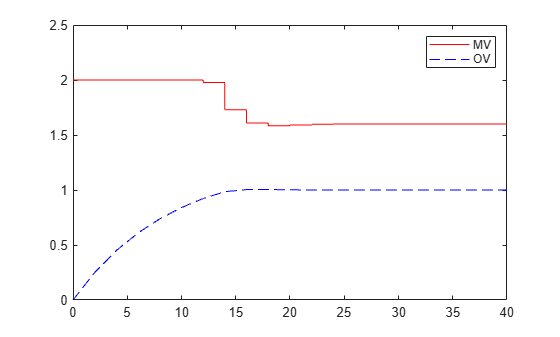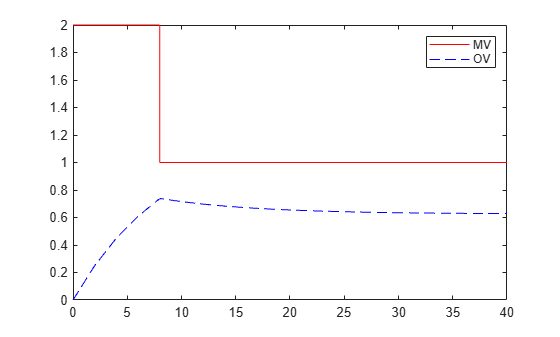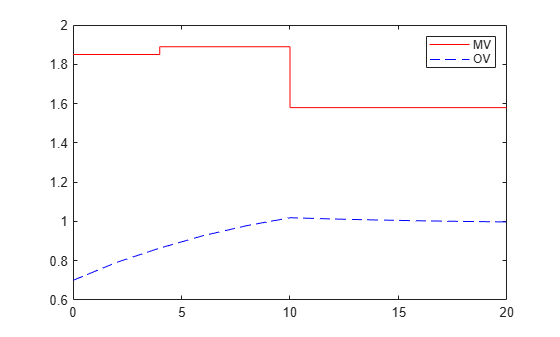# mpcmove

Compute optimal control action and update controller states

## Syntax

``mv = mpcmove(MPCobj,xc,ym,r,v)``
``[mv,info] = mpcmove(MPCobj,xc,ym,r,v)``
``[___] = mpcmove(___,options)``

## Description

Use this command to simulate an MPC controller in closed-loop with a plant model. Call `mpcmove` repeatedly in a for loop to calculate the manipulated variable and update the controller states at each time step.

example

````mv = mpcmove(MPCobj,xc,ym,r,v)` returns the optimal move `mv` and updates the states xc of the controller `MPCobj`.The manipulated variable `mv` at the current time is calculated given: the controller object, `MPCobj`,a pointer to the current estimated extended state, `xc`,the measured plant outputs, `ym`,the output references, `r`,and the measured disturbance input, `v`. If `ym`, `r` or `v` is specified as `[]`, or if it is missing as a last input argument, `mpcmove` uses the appropriate `MPCobj.Model.Nominal` value instead.When using default state estimation, `mpcmove` also updates the controller state referenced by the handle object `xc`. Therefore, when using default state estimation, `xc` always points to the updated controller state. When using custom state estimation, you should update `xc` prior to each `mpcmove` call.```

example

````[mv,info] = mpcmove(MPCobj,xc,ym,r,v)` returns additional information about the optimization problem solved to calculate `mv`.```
````[___] = mpcmove(___,options)` overrides default constraints and weights in `MPCobj` with the values specified in `Options`, an `mpcmoveopt` object. Use `Options` to provide run-time adjustment of constraints and weights during the closed-loop simulation.```

## Examples

collapse all

Perform closed-loop simulation of a plant with one MV and one measured OV.

Define a plant model and create a model predictive controller with MV constraints.

```ts = 2; Plant = ss(0.8,0.5,0.25,0,ts); mpcobj = mpc(Plant);```
```-->The "PredictionHorizon" property is empty. Assuming default 10. -->The "ControlHorizon" property is empty. Assuming default 2. -->The "Weights.ManipulatedVariables" property is empty. Assuming default 0.00000. -->The "Weights.ManipulatedVariablesRate" property is empty. Assuming default 0.10000. -->The "Weights.OutputVariables" property is empty. Assuming default 1.00000. ```
```mpcobj.MV(1).Min = -2; mpcobj.MV(1).Max = 2;```

Obtain an handle object pointing to the controller state.

`xc = mpcstate(mpcobj)`
```-->Assuming output disturbance added to measured output channel #1 is integrated white noise. -->The "Model.Noise" property is empty. Assuming white noise on each measured output. MPCSTATE object with fields Plant: 0 Disturbance: 0 Noise: [1x0 double] LastMove: 0 Covariance: [2x2 double] ```

The controller has one state for the internal plant model, one for the disturbance model, and one to hold the last value of the manipulated variable. All these three states are initialized to zero.

Set the reference signal. There is no measured disturbance.

`r = 1;`

Simulate the closed-loop response by calling `mpcmove` iteratively. In the simulation, assume that the simulated plant is identical to the predictive model. Therefore the plant state `x` in this case is identical to `xc.Plan`t and the plant output is y = `C*x` `+ D*u `= `0.25*x` = `0.25*xc.Plan`t. Here, `mpcmove` updates the controller state referenced by `xc` (therefore including `xc.Plant`), and returns the manipulated variable in `u(i)`, which is used just for plotting.

```t = 0:ts:40; N = length(t); y = zeros(N,1); u = zeros(N,1); for i = 1:N y(i) = 0.25*xc.Plant; u(i) = mpcmove(mpcobj,xc,y(i),r); end```

Analyze the result.

```[ts,us] = stairs(t,u); plot(ts,us,'r-',t,y,'b--') legend('MV','OV')```Modify the MV upper bound as the simulation proceeds using an `mpcmoveopt` object. Since the options argument overrides selected `mpcobj` properties, specify MV constraints again.

```MPCopt = mpcmoveopt; MPCopt.MVMin = -2; MPCopt.MVMax = 2;```

Simulate the closed-loop response and introduce the real-time upper limit change at eight seconds (the fifth iteration step).

```xc = mpcstate(mpcobj); y = zeros(N,1); u = zeros(N,1); for i = 1:N y(i) = 0.25*xc.Plant; if i == 5 MPCopt.MVMax = 1; end u(i) = mpcmove(mpcobj,xc,y(i),r,[],MPCopt); end```

Analyze the results.

```[ts,us] = stairs(t,u); plot(ts,us,'r-',t,y,'b--') legend('MV','OV')```Define a plant model.

```ts = 2; Plant = ss(0.8,0.5,0.25,0,ts);```

Create a model predictive controller with constraints on both the manipulated variable and the rate of change of the manipulated variable. The prediction horizon is `10` intervals, and the control horizon is blocked.

`MPCobj = mpc(Plant,ts,10,[2 3 5]);`
```-->The "Weights.ManipulatedVariables" property is empty. Assuming default 0.00000. -->The "Weights.ManipulatedVariablesRate" property is empty. Assuming default 0.10000. -->The "Weights.OutputVariables" property is empty. Assuming default 1.00000. ```
```MPCobj.MV(1).Min = -2; MPCobj.MV(1).Max = 2; MPCobj.MV(1).RateMin = -1; MPCobj.MV(1).RateMax = 1;```

Initialize (and return an handle to) the controller internal state for simulation.

`xc = mpcstate(MPCobj);`
```-->Assuming output disturbance added to measured output channel #1 is integrated white noise. -->The "Model.Noise" property is empty. Assuming white noise on each measured output. ```
```xc.Plant = 2.8; xc.LastMove = 0.85;```

Compute the optimal control move at the current time.

```y = 0.25*xc.Plant; r = 1; [u,Info] = mpcmove(MPCobj,xc,y,r);```

Analyze the predicted optimal sequences.

```[ts,us] = stairs(Info.Topt,Info.Uopt); plot(ts,us,'r-',Info.Topt,Info.Yopt,'b--') legend('MV','OV')````plot` ignores `Info.Uopt(end)` as it is `NaN`.

Examine the optimal cost.

`Info.Cost`
```ans = 0.0793 ```

## Input Arguments

collapse all

Model predictive controller, specified as an MPC controller object. To create an MPC controller, use `mpc`.

Handle object pointing to current controller state, specified as an `mpcstate` object.

Note

Since `xc` is an handle object, if you copy it to a new variable, the new variable still points to the current state of the same `mpc` object. If this state changes, this is reflected in both `xc` and its copy. To preserve the original state for later use, you can save `xc` to a MAT-file. During simulation, `mpcmove` also updates `xc` when a new control move is calculated. For more information about handle objects, see Handle Object Behavior.

Before you begin a simulation with `mpcmove`, initialize the controller, and return an handle to its state using ```xc = mpcstate(MPCobj)```. Then, modify the default properties of `xc` as appropriate. `mpcmove` modifies the controller state. The handle object `xc` always reflect the current (updated) state of the controller.

If you are using default state estimation, `mpcmove` expects `xc` to represent `xc[n|n-1]`. The `mpcmove` command updates the state values in the previous control interval with that information. Therefore, you should not programmatically update `xc` at all. The default state estimator employs a steady-state Kalman filter.

If you are using custom state estimation, `mpcmove` expects `xc` to represent `xc[n|n]`. Therefore, prior to each `mpcmove` command, you must set `xc.Plant`, `xc.Disturbance`, and `xc.Noise` to the best estimates of these states (using the latest measurements) at the current control interval.

Current measured output values at time k, specified as a column vector of length Nym, where Nym is the number of measured outputs.

If you are using custom state estimation, set `ym = []`.

Plant output reference values, specified as a p-by-Ny array, where p is the prediction horizon of `MPCobj` and Ny is the number of outputs. Row `r(i,:)` defines the reference values at step i of the prediction horizon.

`r` must contain at least one row. If `r` contains fewer than p rows, `mpcmove` duplicates the last row to fill the p-by-Ny array. If you supply exactly one row, therefore, a constant reference applies for the entire prediction horizon.

To implement reference previewing, which can improve tracking when a reference varies in a predictable manner, `r` must contain the anticipated variations, ideally for p steps.

Current and anticipated measured disturbances, specified as a (p+1)-by-Nmd array, where p is the prediction horizon of `MPCobj` and Nmd is the number of measured disturbances. The first row of `v` specifies the current measured disturbance values. Row `v(i+1,:)` defines the anticipated disturbance values at step i of the prediction horizon.

Modeling of measured disturbances provides feedforward control action. If your plant model does not include measured disturbances, use `v` = `[]`.

If your model includes measured disturbances, `v` must contain at least one row. If `v` contains fewer than p+1 rows, `mpcmove` duplicates the last row to fill the (p+1)-by-Nmd array. If you supply exactly one row, a constant measured disturbance applies for the entire prediction horizon.

To implement disturbance previewing, which can improve tracking when a disturbance varies in a predictable manner, `v` must contain the anticipated variations, ideally for p steps.

Run-time options, specified as an `mpcmoveopt` object. Use `options` to override selected properties of `MPCobj` during simulation. These options apply to the current `mpcmove` time instant only. Using `options` yields the same result as redefining or modifying `MPCobj` before each call to `mpcmove`, but involves considerably less overhead. Using `options` is equivalent to using an MPC Controller Simulink® block in combination with optional input signals that modify controller settings, such as MV and OV constraints.

## Output Arguments

collapse all

Optimal manipulated variable moves, returned as a column vector of length Nmv, where Nmv is the number of manipulated variables.

If the controller detects an infeasible optimization problem or encounters numerical difficulties in solving an ill-conditioned optimization problem, `mv` remains at its most recent successful solution, `xc.LastMove`.

Otherwise, if the optimization problem is feasible and the solver reaches the specified maximum number of iterations without finding an optimal solution, `mv`:

• Remains at its most recent successful solution if the `Optimizer.UseSuboptimalSolution` property of the controller is `false`.

• Is the suboptimal solution reached after the final iteration if the `Optimizer.UseSuboptimalSolution` property of the controller is `true`. For more information, see Suboptimal QP Solution.

Solution details, returned as a structure with the following fields.

Predicted optimal manipulated variable adjustments (moves), returned as a (p+1)-by-Nmv array, where p is the prediction horizon and Nmv is the number of manipulated variables.

`Uopt(i,:)` contains the calculated optimal values at time `k+i-1`, for `i = 1,...,p`, where `k` is the current time. The first row of `Info.Uopt` contains the same manipulated variable values as output argument `mv`. Since the controller does not calculate optimal control moves at time `k+p`, `Uopt(p+1,:)` is equal to `Uopt(p,:)`.

Optimal output variable sequence, returned as a (p+1)-by-Ny array, where p is the prediction horizon and Ny is the number of outputs.

The first row of `Info.Yopt` contains the calculated outputs at time `k` based on the estimated states and measured disturbances; it is not the measured output at time `k`. `Yopt(i,:)` contains the predicted output values at time `k+i-1`, for ```i = 1,...,p+1```.

`Yopt(i,:)` contains the calculated output values at time `k+i-1`, for `i = 2,...,p+1`, where `k` is the current time. `Yopt(1,:)` is computed based on the estimated states and measured disturbances.

Optimal prediction model state sequence, returned as a (p+1)-by-Nx array, where p is the prediction horizon and Nx is the number of states in the plant and unmeasured disturbance models (states from noise models are not included).

`Xopt(i,:)` contains the calculated state values at time `k+i-1`, for `i = 2,...,p+1`, where `k` is the current time. `Xopt(1,:)` is the same as the current states state values.

Time intervals, returned as a column vector of length p+1. `Topt(1)` = 0, representing the current time. Subsequent time steps `Topt(i)` are given by `Ts*(i-1)`, where `Ts = MPCobj.Ts` is the controller sample time.

Use `Topt` when plotting the `Uopt`, `Xopt`, or `Yopt` sequences.

Slack variable, ε, used in constraint softening, returned as `0` or a positive scalar value.

• ε = 0 — All constraints were satisfied for the entire prediction horizon.

• ε > 0 — At least one soft constraint is violated. When more than one constraint is violated, ε represents the worst-case soft constraint violation (scaled by your ECR values for each constraint).

Number of solver iterations, returned as one of the following:

• Positive integer — Number of iterations needed to solve the optimization problem that determines the optimal sequences.

• `0` — Optimization problem could not be solved in the specified maximum number of iterations.

• `–1` — Optimization problem was infeasible. An optimization problem is infeasible if no solution can satisfy all the hard constraints.

• `–2` — Numerical error occurred when solving the optimization problem.

Optimization solution status, returned as one of the following:

• `'feasible'` — Optimal solution was obtained (`Iterations` > 0)

• `'infeasible'` — Solver detected a problem with no feasible solution (`Iterations` = –1) or a numerical error occurred (`Iterations` = –2)

• `'unreliable'` — Solver failed to converge (`Iterations` = 0). In this case, if `MPCobj.Optimizer.UseSuboptimalSolution` is `false`, `u` freezes at the most recent successful solution. Otherwise, it uses the suboptimal solution found during the last solver iteration.

Objective function cost, returned as a nonnegative scalar value. The cost quantifies the degree to which the controller has achieved its objectives. For more information, see Optimization Problem.

The cost value is only meaningful when ```QPCode = 'feasible'```, or when `QPCode = 'feasible'` and `MPCobj.Optimizer.UseSuboptimalSolution` is `true`.

## Tips

• `mpcmove` updates `xc`, even though it is an input argument.

• If `ym`, `r` or `v` is specified as `[]`, or if it is missing as a last input argument, `mpcmove` uses the appropriate `MPCobj.Model.Nominal` value instead.

• To view the predicted optimal behavior for the entire prediction horizon, plot the appropriate sequences provided in `Info`.

• To determine the optimization status, check `Info.Iterations` and `Info.QPCode`.

## Version History

Introduced before R2006a